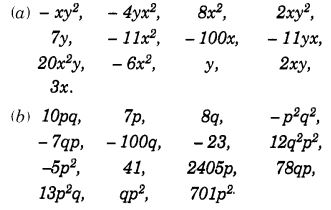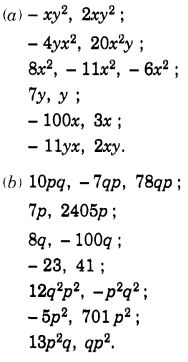NCERT Solutions for Class 7 Maths Chapter 12 Algebraic Expressions Ex 12.1 are part of NCERT Solutions for Class 7 Maths. Here we have given NCERT Solutions for Class 7 Maths Chapter 12 Algebraic Expressions Ex 12.1.

 Board CBSE Textbook NCERT Class Class 7 Subject Maths Chapter Chapter 12 Chapter Name Algebraic Expressions Exercise Ex 12.1 Number of Questions Solved 7 Category NCERT Solutions

## NCERT Solutions for Class 7 Maths Chapter 12 Algebraic Expressions Ex 12.1

Question 1.
Get the algebraic expressions in the following cases using variables, constants, and arithmetic operations.
(i) Subtraction of z from y.
Solution:
y – z

(ii) One-half of the sum of numbers x and y.
Solution:
$$\frac{1}{2}$$  (x -y)

(iii) The number z multiplied by itself.
Solution:
z × z i.e., z2

(iv) One-fourth of the product of numbers p and q.
Solution:
$$\frac{1}{4}$$ pq

(v) Numbers x and y both squared and added.
Solution:
x2 + y2

(vi) Number 5 added to three times the product of numbers m and n.
Solution:
3mn + 5

(vii) Product of numbers y and z subtracted from 10.
Solution:
10 – yz

(viii) Sum of numbers a and 6 subtracted from their product.
Solution:
ab – (a + b)

Question 2.
(i) Identify the terms and their factors in the following expressions. Show the terms and factors by tree diagrams :

(a) x – 3
(b) 1 + x + x2
(c) y – y3
(d) 5xy2 + 7x2y
(e) -ab + 2b2 -3a2

(ii) Identify terms and factors in the expressions given below :

(a) – 4x + 5
(b) – 4x + 5y
(c) 5y + 3y2
(d) xy + 2x2y2
(e) pq + q
(f) 1.2 ab -2.4 b + 3.6 a
(g) $$\frac { 3 }{ 4 }$$ x + $$\frac { 1 }{ 4 }$$
(h) 0.1 p2 + 0.2 q2

Solution: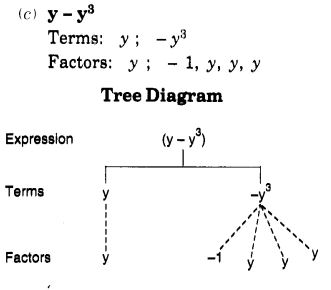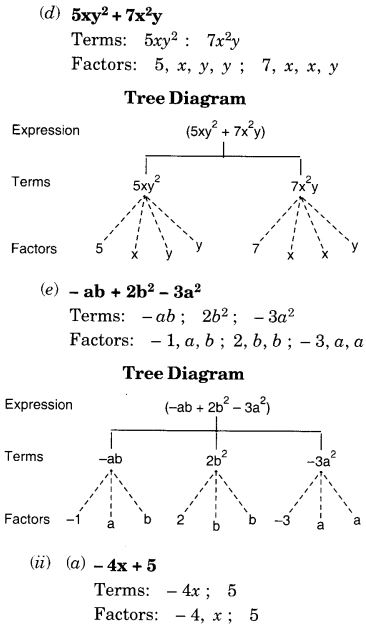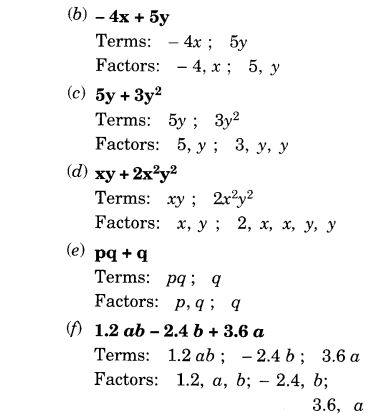Question 3.
Identify the numerical coefficients of terms (other than constants) in the following expressions :

1. 5 – 3t2
2. 1 + t + t2 + t3
3. x + 2xy + 3y
4. 100m + 1000n
5. -p2q2 + 7pq
6. 1.2 a + 0.8 b
7. 3.14 r2
8. 2 (l + b)
9. 0.1 y + 0.01 y2.

Solution: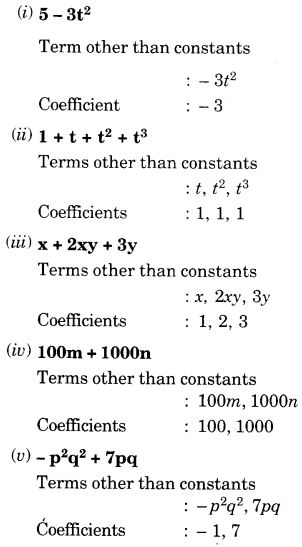Question 4.
(a) Identify terms which contain x and give the coefficient of x.

1. y2x + y
2. 13y2 – 8yx
3. x + y + 2
4. 5 + z + zx
5. 1 + x + xy
6. 12xy2 + 25
7. 7x + xy2.

(b) Identify terms which contain y2 and give the coefficient of y2.

1. 8 – xy2
2. 5y2 + 7x
3. 2x2y – 15xy2 + 7y2

Solution: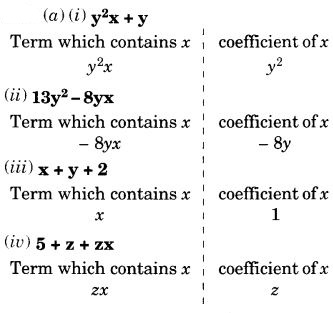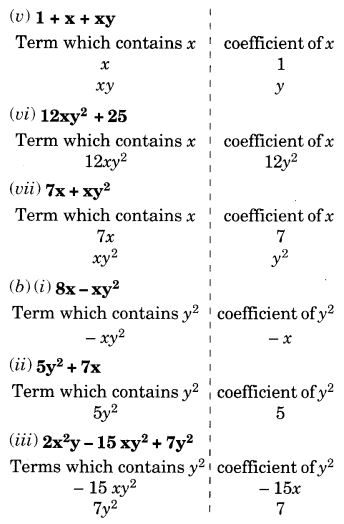Question 5.
Classify into monomials, binomials and trinomials.

1. 4y – 7z
2. y2
3. x + y – xy
4. 100
5. ab – a – b
6. 5 – 3t
7. 4p2q – 4pq2
8. 7mn
9. z2 – 3z + 8 a2 + b2
10. z2 + z
11. 1 + x+ x2

Solution:
We know that an algebraic expression containing only one term is called a monomial. So, the monomials are : (ii), (iv), and (viii).

We know that an algebraic expression containing two terms is called a binomial. So, the binomials are : (i), (vi), (vii), (x) and (xi).

We know that an algebraic expression containing three terms is called a trinomial. So, the trinomial are : (iii), (v), (ix) and (xii).

Question 6.
State whether a given pair of terms is of like or unlike terms :
(i) 1, 100
Solution:
Like

(ii) -7x, $$\frac{5}{2}$$x
Solution:
Like

(iii) – 29x, – 29y
Solution:
Unlike

(iv)14xy, 42yx
Solution:
Like

(v) 4m2p, 4mp2
Unlike

(vi) 12xz, 12x2z2
Solution:
Unlike

(i) 4y – 7z.
This expression is a binomial because it contains two terms: 4y and – Iz.
(ii) y2.
This expression is a monomial because it contains only one term: y2
(iii) x + y – xy.
This expression is a trinomial because it contains three terms: x, y, and – xy.
(iv) 100.
This expression is a monomial because it contains only one term: 100
(v) ab – a – b.
This expression is a trinomial because it contains three terms: ab, -a, and -b
(vi) 5 – 3t.
This expression is a binomial because it contains two terms : 5 and – 31.
(vii) 4p2q – 4pq2.
This expression is a binomial because it contains two terms: 4p2q and – 4pq2.
(viii) 7mn.
This expression is a monomial because it contains only one term : 7mn.
(ix) z2 – 3z + 8.
This expression is a trinomial because it contains three terms : z2, – 3z and 8.
(x) a2 + b2.
This expression is a binomial because it contains two terms: a2 and b2.
(xi) z2 + z.
This expression is a binomial because it contains two terms : z2 and z.
(xii) 1 + x + x2.
This expression is a trinomial because it contains three terms: 1, x, and x2.

Question 6.
State whether a given pair of terms is of like or unlike terms :
(i) 1, 100
Solution:
Like

(ii) -7x, $$\frac{5}{2}$$x
Solution:
Like

(iii) – 29x, – 29y
Solution:
Unlike

(iv)14xy, 42yx
Solution:
Like

(v) 4m2p, 4mp2